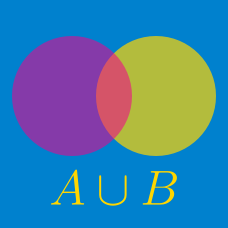Probability

# Sets - Relative Complement

If $A$ and $B$ are subsets of the set $U=\{0,3,5,10,12,16,18\}$ such that $A \cap B^{C}=\{3,5\}, A^{C} \cap B=\{10,12\}, A^{C} \cap B^{C}=\{16,18\},$ then which of the following is equal to $A \cap B?$

Details and assumptions

You may choose to read the summary page Set Notation.

Consider the ground set $U=\{x \mid x \mbox{ is a positive integer } \leq 17\}.$ If $A=\{1,2,\ldots,5\}$ and $B$ is a subset of $U$ such that $A \cap B=\{4, 5\}, (U \setminus A) \cap (U \setminus B)=\{16, 17\},$ what is $\lvert B \rvert?$

Consider the ground set $U=\{x \mid x \mbox{ is a positive integer } \leq 35\}$ and let $A$ and $B$ be two subsets of $U$ such that $A=\{1, 2, 3, \ldots, 17\}, \; A \setminus(A \setminus B)=A.$ What is $\lvert (U \backslash A) \cup B \rvert?$

Details and assumptions

You may choose to read the summary page Set Notation.

Consider the sets $A=\{x \mid 3 \leq x < 50\}, B=\{x \mid x \leq 0 \text{ or } x > 43\}.$ How many integers are there in the set $A \setminus B?$

Details and assumptions

You may choose to read the summary page Set Notation.

Consider a ground set $U$ and let $A$ and $B$ be two subsets of $U.$ If $\lvert U \rvert=70, \lvert A \rvert=37, \lvert A \cap B \rvert=13, \lvert A \cup B \rvert=48,$ what is $\lvert U \setminus B^c \rvert?$

Details and assumptions

You may choose to read the summary page Set Notation.

×